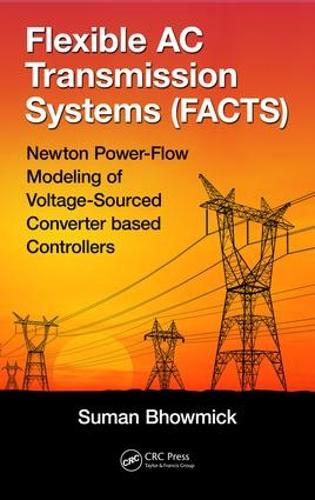•Flexible AC Transmission Systems (FACTS): Newton Power-Flow Modeling of Voltage-Sourced Converter-Based Controllers (Hardback)

(author)
£105.00
Hardback 291 Pages / Published: 10/05/2016
• We can order this

Usually dispatched within 3 weeks

Flexible AC Transmission Systems (FACTS): Newton Power-Flow Modeling of Voltage-Sourced Converter-Based Controllers introduces different voltage-sourced converter (VSC)-based FACTS controllers and VSC-based high-voltage direct current (VSC-HVDC) systems and their working principles, explaining how FACTS controllers exchange real and reactive power with systems.

Subsequently, the book:

Describes the Newton-Raphson method and its application for solving the power-flow problemPresents the Newton power-flow modeling of the static synchronous series compensator (SSSC), unified power-flow controller (UPFC), interline power-flow controller (IPFC), generalized unified power-flow controller (GUPFC), and static synchronous compensator (STATCOM), accommodating the practical device constraint limits (because of the unique modeling strategy, the existing Newton power-flow codes can be reused)Develops a unified Newton power-flow model of AC systems incorporating multiterminal VSC-HVDC systems with pulse-width modulation (PWM) control schemes, directly yielding the VSC modulation indices from the power-flow solutionProvides numerous case studies for validation of Newton power-flow models, elaborating on the occurrences and checking of unrealistic power-flow solutions in isolated casesIncludes detailed derivations of all the difficult formulae as well as solved problems on typical VSC-based FACTS controllers

Flexible AC Transmission Systems (FACTS): Newton Power-Flow Modeling of Voltage-Sourced Converter-Based Controllers assumes at least an undergraduate-level understanding of engineering mathematics, network analysis, electrical machines, electrical power systems, and power electronics. Thus, the book provides a valuable reference for practitioners as well as senior-undergraduate and graduate students in electrical engineering and electrical power systems.

Publisher: Taylor & Francis Inc
ISBN: 9781498756198
Number of pages: 291
Weight: 590 g
Dimensions: 235 x 156 x 23 mm